Question

# Giving a test to a group of students, the grades and gender are summarized below Male...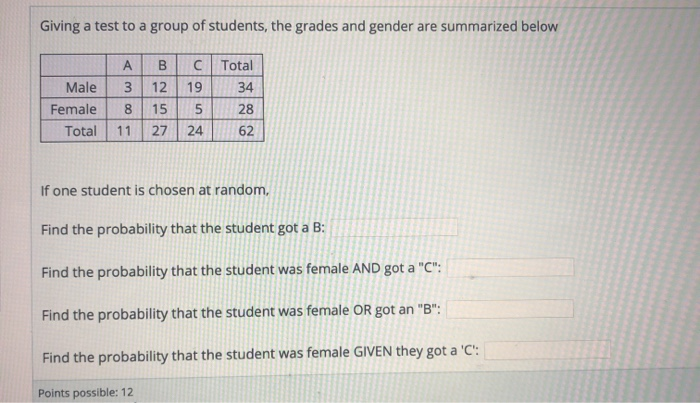Giving a test to a group of students, the grades and gender are summarized below Male Female Total A B 3 12 8 15 11 27 C Total 19 34 5 28 24 62 If one student is chosen at random, Find the probability that the student got a B: Find the probability that the student was female AND got a "C": Find the probability that the student was female OR got an "B": Find the probability that the student was female GIVEN they got a 'c': Points possible: 12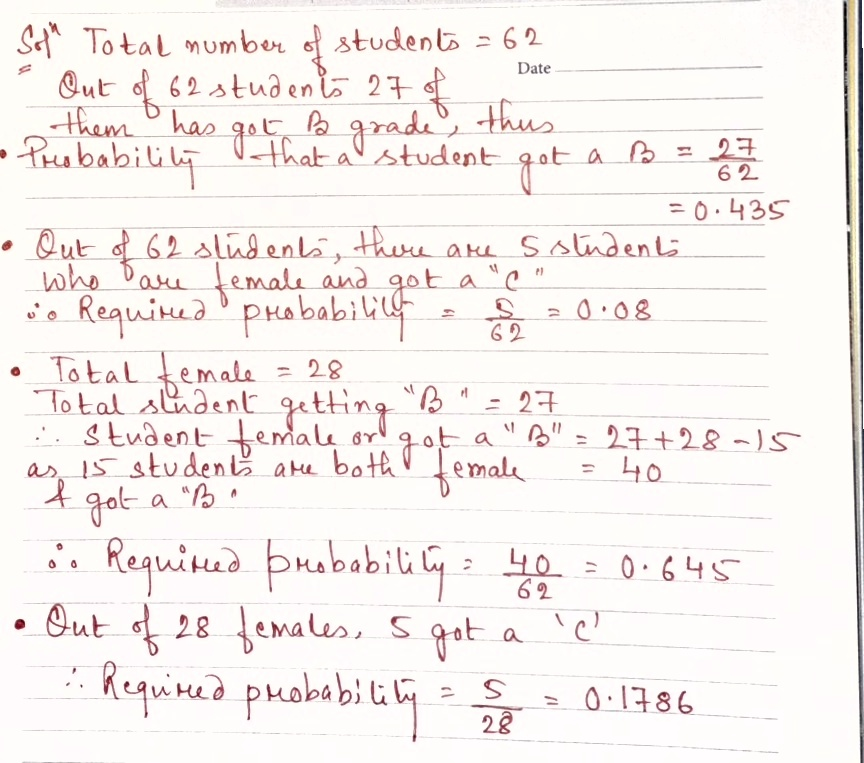#### Earn Coins

Coins can be redeemed for fabulous gifts.

Similar Homework Help Questions
• ### Giving a test to a group of students, the grades and gender are summarized below A...

Giving a test to a group of students, the grades and gender are summarized below A B C Total Male 4 10 11 25 Female 12 8 17 37 Total 16 18 28 62 If one student is chosen at random, Find the probability that the student was male:     Find the probability that the student was female AND got a "B":     Find the probability that the student was male OR got an "B":     If one student is chosen at random,...

• ### Giving a test to a group of students, the grades and gender are summarized below A...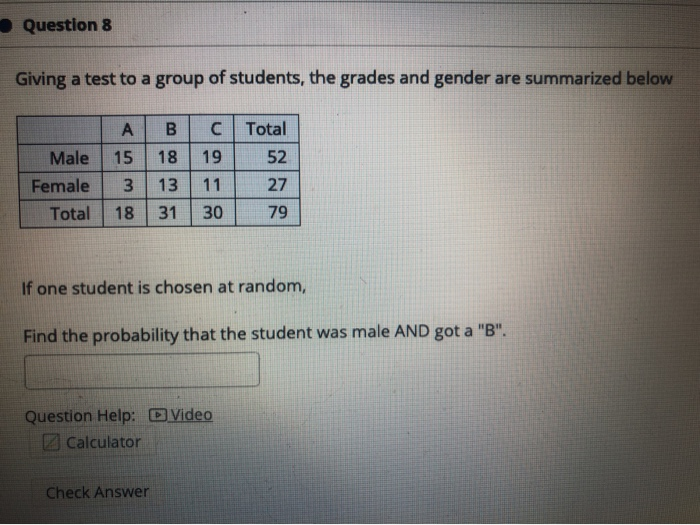Giving a test to a group of students, the grades and gender are summarized below A B C Total Male 15 18 19 52 Female 3 13 11 27 Total 18 31 30 79 If one student is chosen at random, Find the probability that the student was male AND got a "B".    Question 8 Giving a test to a group of students, the grades and gender are summarized below A B Total 52 18 Male Female Total 15...

• ### Giving a test to a group of students, the grades and gender are summarized below A...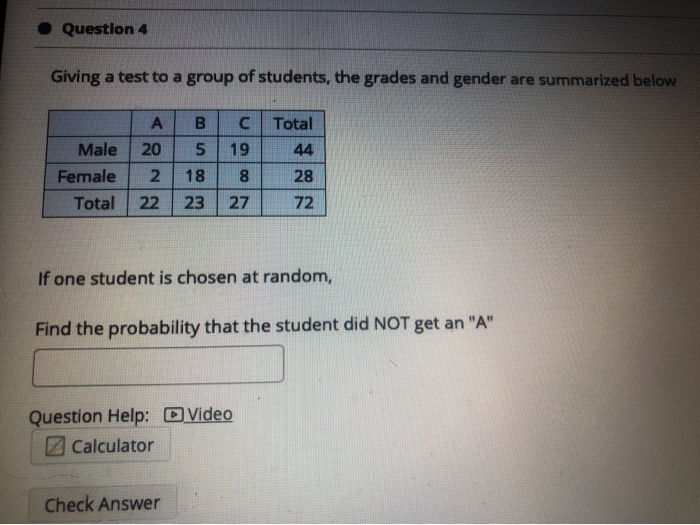Giving a test to a group of students, the grades and gender are summarized below A B C Total Male 20 5 19 44 Female 2 18 8 28 Total 22 23 27 72 If one student is chosen at random, Find the probability that the student did NOT get an "A"    Question 4 Giving a test to a group of students, the grades and gender are summarized below B А 20 C Total 19 44 5 Male Female...

• ### Giving a test to a group of students, the grades and gender are summarized below A...

Giving a test to a group of students, the grades and gender are summarized below A B C Total Male 12 2 16 30 Female 6 7 18 31 Total 18 9 34 61 If one student is chosen at random, Find the probability that the student was female OR got an "A". A test was given to a group of students. The grades and gender are summarized below A B C Total Male 2 3 18 23 Female 16...

• ### Giving a test to a group of students, the grades and gender are summarized below Male...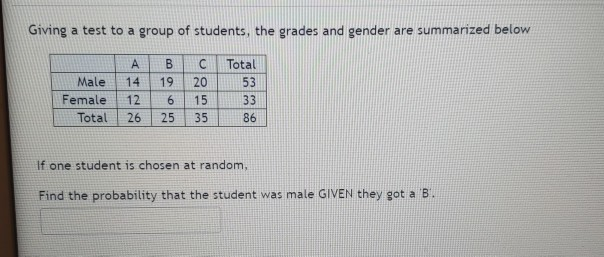Giving a test to a group of students, the grades and gender are summarized below Male Female Total ABC Total 14 19 20 12 6 15 26 25 35 If one student is chosen at random, Find the probability that the student was male GIVEN they got a B.

• ### Giving a test to a group of students, the grades and gender are summarized below Grades...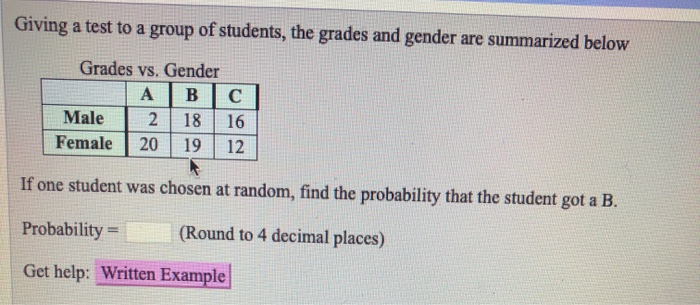Giving a test to a group of students, the grades and gender are summarized below Grades vs. Gender A | B | C Male 2 | 18 | 16 Female 2019 12 If one student was chosen at random, find the probability that the student got a B. Probability = (Round to 4 decimal places) Get help: Written Example Giving a test to a group of students, the grades and gender are summarized below Grades vs. Gender ABC Male 13...

• ### Giving a test to a group of students, the grades and gender are summarized below Male...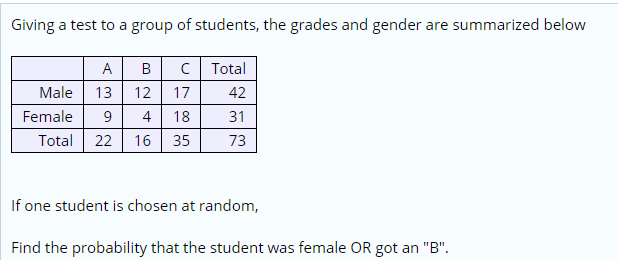Giving a test to a group of students, the grades and gender are summarized below Male Female Total A 13 9 22 B 12 4 16 C 17 18 35 Total 42 31 73 If one student is chosen at random, Find the probability that the student was female OR got an "B".

• ### Giving a test to a group of students, the grades and gender are summarized below В....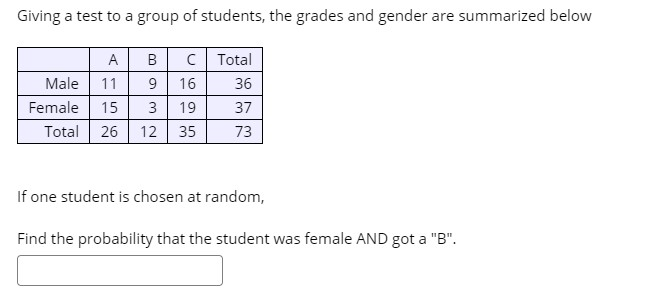Giving a test to a group of students, the grades and gender are summarized below В. А. Male 11 Female 15 Total 26 C Total 16 36 19 37 35 73 12 If one student is chosen at random, Find the probability that the student was female AND got a "B".

• ### Giving a test to a group of students, the grades and gender are summarized below A...

Giving a test to a group of students, the grades and gender are summarized below A B C Total Male 13 2 7 22 Female 15 18 16 49 Total 28 20 23 7 If one student is chosen at random, Find the probability that the student was male OR got an "A".

• ### A test was given to a group of students. The grades and gender are summarized below...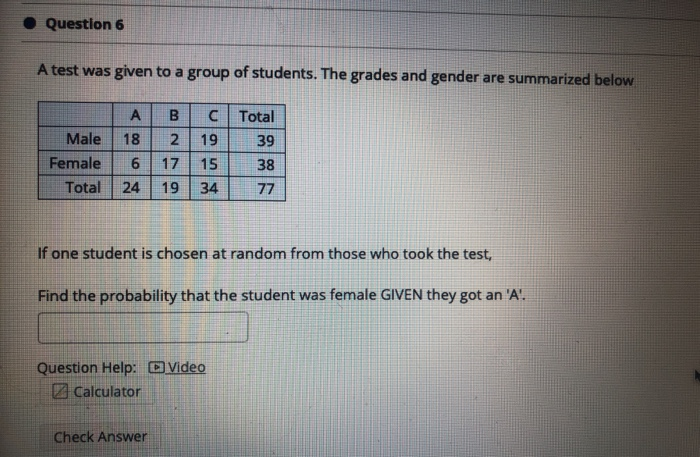A test was given to a group of students. The grades and gender are summarized below A B C Total Male 18 2 19 39 Female 6 17 15 38 Total 24 19 34 77 If one student is chosen at random from those who took the test, Find the probability that the student was female GIVEN they got an 'A'.    Question 6 A test was given to a group of students. The grades and gender are summarized below...